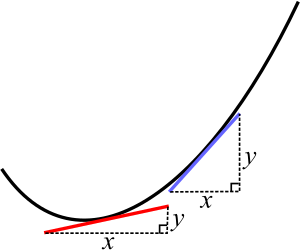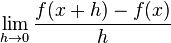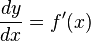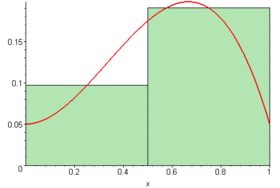# Calculus facts for kids

Kids Encyclopedia Facts

Calculus is a branch of mathematics which helps us understand changes between values that are related by a function. For example, if you had one formula telling how much money you got every day, calculus would help you understand related formulas like how much money you have in total, and whether you are getting more money or less than you used to. All these formulas are functions of time, and so that is one way to think of calculus—studying functions of time. There are two different types of calculus. Differential calculus divides things into small (different) pieces and tells us how they change from one moment to the next, while integral calculus joins (integrates) the small pieces together and tells us how much of something is made, overall, by a series of changes. Calculus is used in many different areas of study such as physics, astronomy, biology, engineering, economics, medicine and sociology.

## History

In the 1670s and 1680s, Sir Isaac Newton in England and Gottfried Leibniz in Germany figured out calculus at the same time, working separately from each other. Newton wanted to have a new way to predict where to see planets in the sky, because astronomy had always been a popular and useful form of science, and knowing more about the motions of the objects in the night sky was important for navigation of ships. Leibniz wanted to measure the space (area) under a curve (a line which is not straight). Many years later, the two men argued over who discovered it first. Scientists from England supported Newton, but scientists from the rest of Europe supported Leibniz. Most mathematicians today agree that both men share the credit equally. Some parts of modern calculus come from Newton, such as its uses in physics. Other parts come from Leibniz, such as the symbols used to write it.

They were not the first people to use mathematics to describe the physical world—Aristotle and Pythagoras came earlier, and so did Galileo, who said that mathematics was the language of science. But they were the first to design a system that describes how things change over time and can predict how they will change in the future.

The name "calculus" was the Latin word for a small stone the ancient Romans used in counting and gambling. The English word "calculate" comes from the same Latin word.

## Differential calculus

Differential calculus is the process of finding out the rate of change of a variable compared to another variable. It can be used to find the speed of a moving object or the slope of a curve, figure out the maximum or minimum points of a curve, or find answers to problems in the electricity and magnetism areas of physics, among many other uses.

Many amounts can be variables, which can change their value unlike numbers such as 5 or 200. Some examples of variables are distance and time. The speed of an object is how far it travels in a particular time. So if a town is 80 kilometres (50 miles) away and a person in a car gets there in one hour, they have traveled at an average speed of 80 kilometres (50 miles) per hour. But this is only an average—they may have been traveling faster at some times (on a highway) and slower at others (at a traffic light or on a small street where people live). Imagine a driver trying to figure out a car's speed using only its odometer (distance meter) and clock, without a speedometer!

Until calculus was invented, the only way to work this out was to cut the time into smaller and smaller pieces, so the average speed over the smaller time would get closer and closer to the actual speed at a point in time. This was a very long and hard process and had to be done each time people wanted to work something out.On a curve, two different points have different slopes. The red and blue lines are tangents to the curve.

A very similar problem is to find the slope (how steep it is) at any point on a curve. The slope of a straight line is easy to work out—it is simply how much it goes up (y or vertical) divided by how much it goes across (x or horizontal). On a curve, though, the slope is a variable (has different values at different points) because the line bends. But if the curve was to be cut into very, very small pieces, the curve at the point would look almost like a very short straight line. So to work out its slope, a straight line can be drawn through the point with the same slope as the curve at that point. If it is done exactly right, the straight line will have the same slope as the curve, and is called a tangent. But there is no way to know (without very complicated mathematics) whether the tangent is exactly right, and our eyes are not accurate enough to be certain whether it is exact or simply very close.

What Newton and Leibniz found was a way to work out the slope (or the speed in the distance example) exactly using simple and logical rules. They divided the curve into an infinite number of very small pieces. They then chose points on either side of the range they were interested in and worked out tangents at each. As the points moved closer together towards the point they were interested in, the slope approached a particular value as the tangents approached the real slope of the curve. They said that this particular value it approached was the actual slope.

Let's say we have a function y = f(x). f is short for function, so this equation means "y is a function of x". This tells us that how high y is on the vertical axis depends on what x (the horizontal axis) is at that time. For example, with the equation y = x², we know that if x is 1, then y will be 1; if x is 3, then y will be 9; if x is 20, then y will be 400.$\lim_{h\rightarrow0} \frac{f(x+h) - f(x)}{h}$

If we use y = x², the derivative produced using this method is 2x, or 2 multiplied by x. So we know without having to draw any tangent lines that at any point on the curve f(x) = x², the derivative f ′(x) (marked with the prime symbol) will be 2x at any point. This process of working out a slope using limits is called differentiation, or finding the derivative.

Leibniz came to the same result, but called h "dx", which means "with respect to x". He called the resulting change in f(x) "dy", which means "a tiny amount of y". Leibniz's notation is used by more books because it is easy to understand when the equations become more complicated. In Leibniz notation:$\frac{dy}{dx} = f'(x)$

Mathematicians have grown this basic theory to make simple algebra rules which can be used to find the derivative of almost any function.

## Main idea of calculus

The main idea in calculus is called the fundamental theorem of calculus. This main idea says that the two calculus processes, differential and integral calculus, are opposites. That is, a person can use differential calculus to undo an integral calculus process. Also, a person can use integral calculus to undo a differential calculus method. This is just like using division to "undo" multiplication, or addition to "undo" subtraction.

In a single sentence, the fundamental theorem runs something like this: "The derivative of the integral of a function f is the function itself".

## Demonstration of main idea of calculus

### How to use integral calculus to find areasWe can approximate the area under a curve by adding up the areas of many rectangles underneath the curve. The more rectangles we use, the better our approximation.

The method integral calculus uses to find areas of shapes is to break the shape up into many small boxes, and add up the area of each of the boxes. This gives an approximation to the area. If the boxes are made narrower and narrower, then there are more and more of them, and the area of all the boxes becomes very close to the area of the shape. One of the main ideas of calculus is that we can imagine having an infinite number of these boxes, each infinitely narrow, and then we would have the exact area of the shape.

## Other uses of calculus

Calculus is used to describe things that change, like things in nature. It can be used for showing and learning all of these:

• How waves move. Waves are very important in the natural world. For example, sound and light can be thought of as waves.
• Where heat moves, like in a house. This is useful for architecture (building houses), so that the house can be as cheap to heat as possible.
• How very small things like atoms act.
• How fast something will fall, also known as gravity.
• How machines work, also known as mechanics.
• The path of the moon as it moves around the earth. Also, the path of the earth as it moves around the sun, and any planet or moon moving around anything in space.